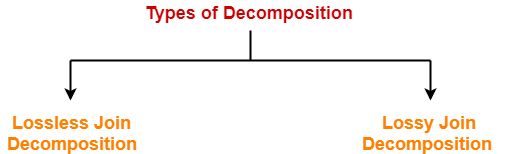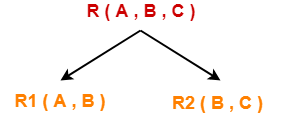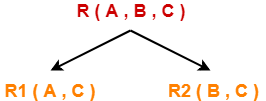# Decomposition in DBMS | Lossless | Lossy

## Decomposition of a Relation-

 The process of breaking up or dividing a single relation into two or more sub relations is called as decomposition of a relation.

## Properties of Decomposition-

The following two properties must be followed when decomposing a given relation-

## 1. Lossless decomposition-

Lossless decomposition ensures-

• No information is lost from the original relation during decomposition.
• When the sub relations are joined back, the same relation is obtained that was decomposed.

Every decomposition must always be lossless.

## 2. Dependency Preservation-

Dependency preservation ensures-

• None of the functional dependencies that holds on the original relation are lost.
• The sub relations still hold or satisfy the functional dependencies of the original relation.

## Types of Decomposition-

Decomposition of a relation can be completed in the following two ways-## 1. Lossless Join Decomposition-

• Consider there is a relation R which is decomposed into sub relations R1 , R2 , …. , Rn.
• This decomposition is called lossless join decomposition when the join of the sub relations results in the same relation R that was decomposed.
• For lossless join decomposition, we always have-

 R1 ⋈ R2 ⋈ R3 ……. ⋈ Rn = R

where ⋈ is a natural join operator

## Example-

Consider the following relation R( A , B , C )-

 A B C 1 2 1 2 5 3 3 3 3

R( A , B , C )

Consider this relation is decomposed into two sub relations R1( A , B ) and R2( B , C )-The two sub relations are-

 A B 1 2 2 5 3 3

R1( A , B )

 B C 2 1 5 3 3 3

R2( B , C )

Now, let us check whether this decomposition is lossless or not.

For lossless decomposition, we must have-

R1 ⋈ R2 = R

Now, if we perform the natural join ( ⋈ ) of the sub relations R1 and R2 , we get-

 A B C 1 2 1 2 5 3 3 3 3

This relation is same as the original relation R.

Thus, we conclude that the above decomposition is lossless join decomposition.

### NOTE-

• Lossless join decomposition is also known as non-additive join decomposition.
• This is because the resultant relation after joining the sub relations is same as the decomposed relation.
• No extraneous tuples appear after joining of the sub-relations.

## 2. Lossy Join Decomposition-

• Consider there is a relation R which is decomposed into sub relations R1 , R2 , …. , Rn.
• This decomposition is called lossy join decomposition when the join of the sub relations does not result in the same relation R that was decomposed.
• The natural join of the sub relations is always found to have some extraneous tuples.
• For lossy join decomposition, we always have-

 R1 ⋈ R2 ⋈ R3 ……. ⋈ Rn ⊃ R

where ⋈ is a natural join operator

## Example-

Consider the following relation R( A , B , C )-

 A B C 1 2 1 2 5 3 3 3 3

R( A , B , C )

Consider this relation is decomposed into two sub relations as R1( A , C ) and R2( B , C )-The two sub relations are-

 A C 1 1 2 3 3 3

R1( A , B )

 B C 2 1 5 3 3 3

R2( B , C )

Now, let us check whether this decomposition is lossy or not.

For lossy decomposition, we must have-

R1 ⋈ R2 ⊃ R

Now, if we perform the natural join ( ⋈ ) of the sub relations R1 and R2 we get-

 A B C 1 2 1 2 5 3 2 3 3 3 5 3 3 3 3

This relation is not same as the original relation R and contains some extraneous tuples.

Clearly, R1 ⋈ R2 ⊃ R.

Thus, we conclude that the above decomposition is lossy join decomposition.

### NOTE-

• Lossy join decomposition is also known as careless decomposition.
• This is because extraneous tuples get introduced in the natural join of the sub-relations.
• Extraneous tuples make the identification of the original tuples difficult.

Next Article- Rules to Determine Lossless and Lossy Decomposition

Get more notes and other study material of Database Management System (DBMS).

Watch video lectures by visiting our YouTube channel LearnVidFun.

SummaryArticle Name
Decomposition in DBMS | Lossless | Lossy
Description
Decomposition in DBMS is a process of dividing a relation into sub relations. Types of Decomposition in DBMS- Lossless Decomposition and Lossy Decomposition.
Author
Publisher Name
Gate Vidyalay
Publisher Logo